# Maharashtra Board Class 11 Physics Solutions Chapter 10 Electrostatics

Balbharti Maharashtra State Board 11th Physics Textbook Solutions Chapter 10 Electrostatics Textbook Exercise Questions and Answers.

## Maharashtra State Board 11th Physics Solutions Chapter 10 Electrostatics

1. Choose the correct option.

Question 1.
A positively charged glass rod is brought close to a metallic rod isolated from the ground. The charge on the side of the metallic rod away from the glass rod will be
(A) same as that on the glass rod and equal in quantity
(B) opposite to that on the glass of and equal in quantity
(C) same as that on the glass rod but lesser in quantity
(D) same as that on the glass rod but more in quantity
(A) same as that on the glass rod and equal in quantity

Question 2.
An electron is placed between two parallel plates connected to a battery. If the battery is switched on, the electron will
(A) be attracted to the +ve plate
(B) be attracted to the -ve plate
(C) remain stationary
(D) will move parallel to the plates
(A) be attracted to the +ve plateQuestion 3.
A charge of + 7 µC is placed at the centre of two concentric spheres with radius 2.0 cm and 4.0 cm respectively. The ratio of the flux through them will be
(A) 1 : 4
(B) 1 : 2
(C) 1 : 1
(D) 1 : 16
(C) 1 : 1

Question 4.
Two charges of 1.0 C each are placed one meter apart in free space. The force between them will be
(A) 1.0 N
(B) 9 × 109 N
(C) 9 × 10-9 N
(D) 10 N
(B) 9 × 109 N

Question 5.
Two point charges of +5 µC are so placed that they experience a force of 80 × 10-3 N. They are then moved apart, so that the force is now 2.0 × 10-3 N. The distance between them is now
(A) 1/4 the previous distance
(B) double the previous distance
(C) four times the previous distance
(D) half the previous distance
(B) double the previous distance

Question 6.
A metallic sphere A isolated from ground is charged to +50 µC. This sphere is brought in contact with other isolated metallic sphere B of half the radius of sphere A. The charge on the two sphere will be now in the ratio
(A) 1 : 2
(B) 2 : 1
(C) 4 : 1
(D) 1 : 1
(D) 1 : 1

Question 7.
Which of the following produces uniform electric field?
(A) point charge
(B) linear charge
(C) two parallel plates
(D) charge distributed an circular any
(C) two parallel plates

Question 8.
Two point charges of A = +5.0 µC and B = -5.0 µC are separated by 5.0 cm. A point charge C = 1.0 µC is placed at 3.0 cm away from the centre on the perpendicular bisector of the line joining the two point charges. The charge at C will experience a force directed towards
(A) point A
(B) point B
(C) a direction parallel to line AB
(D) a direction along the perpendicular bisector
(C) a direction parallel to line AB

Question 1.
What is the magnitude of charge on an electron?
The magnitude of charge on an electron is 1.6 × 10-19 C

Question 2.
State the law of conservation of charge.
In any given physical process, charge may get transferred from one part of the system to another, but the total charge in the system remains constant”
OR
For an isolated system, total charge cannot be created nor destroyed.

Question 3.
Define a unit charge.
Unit charge (one coulomb) is the amount of charge which, when placed at a distance of one metre from another charge of the same magnitude in vacuum, experiences a force of 9.0 × 109 N.

Question 4.
Two parallel plates have a potential difference of 10V between them. If the plates are 0.5 mm apart, what will be the strength of electric charge.
V=10V
d = 0.5 mm = 0.5 × 10-3 m
To find: The strength of electric field (E)
Formula: E = $$\frac {V}{d}$$
Calculation: From formula,
E = $$\frac {10}{0.5×10^{-3}}$$
20 × 103 V/m

Question 5.
What is uniform electric field?
A uniform electric field is a field whose magnitude and direction are same at all points. For example, field between two parallel plates as shown in the diagram.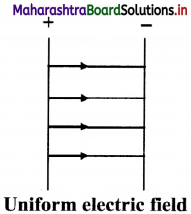Question 6.
If two lines of force intersect of one point. What does it mean?
If two lines of force intersect of one point, it would mean that electric field has two directions at a single point.Question 7.
State the units of linear charge density.
SI unit of λ is (C / m).

Question 8.
What is the unit of dipole moment?
i. Strength of a dipole is measured in terms of a quantity called the dipole moment.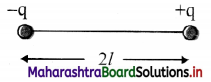ii. Let q be the magnitude of each charge and 2$$\vec{l}$$ be the distance from negative charge to positive charge. Then, the product q(2$$\vec{l}$$) is called the dipole moment $$\vec{p}$$.

iii. Dipole moment is defined as $$\vec{p}$$ = q(2$$\vec{l}$$)

iv. A dipole moment is a vector whose magnitude is q (2$$\vec{l}$$) and the direction is from the negative to the positive charge.

v. The unit of dipole moment is coulomb-metre (C m) or debye (D).

Question 9.
What is relative permittivity?
i. Relative permittivity or dielectric constant is the ratio of absolute permittivity of a medium to the permittivity of free space.
It is denoted as K or εr.
i.e., K or εr = $$\frac {ε}{ε_0}$$

ii. It is the ratio of the force between two point charges placed a certain distance apart in free space or vacuum to the force between the same two point charges when placed at the same distance in the given medium.
i.e., K or εr = $$\frac {F_{vacuum}}{F_{medium}}$$

iii. It is also called as specific inductive capacity or dielectric constant.

3. Solve numerical examples.

Question 1.
Two small spheres 18 cm apart have equal negative charges and repel each other with the force of 6 × 10-8 N. Find the total charge on both spheres.
Solution:
Given: F = 6 × 10-8 N, r = 18 cm = 18 × 10-2 m
To find: Total charge (q1 + q2)
Formula: F = $$\frac {1}{4πε_0}$$ $$\frac {q_1q_2}{r^2}$$
Calculation: From formula,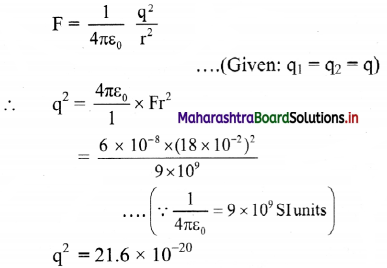Taking square roots from log table,
∴ q = -4.648 × 10-10 C
….(∵ the charges are negative)
Total charge = q1 + q2 = 2q
= 2 × (-4.648) × 10-10
= -9.296 × 10-10 C

Question 2.
A charge + q exerts a force of magnitude – 0.2 N on another charge -2q. If they are separated by 25.0 cm, determine the value of q.
Given: q1 = + q, q2 = -2q, F = -0.2 N
r = 25 cm = 25 × 10-2 m
To find: Charge (q)
Formula: F = $$\frac {1}{4πε_0}$$ $$\frac {q_1q_2}{r^2}$$
Calculation: From formula,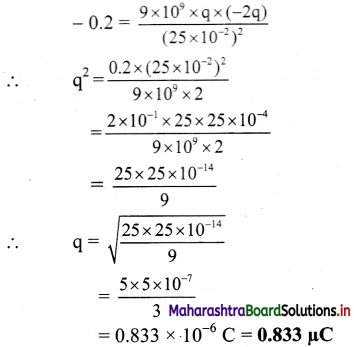[Note: The answer given above is calculated in accordance with textual method considering the given data]Question 3.
Four charges of +6 × 10-8 C each are placed at the corners of a square whose sides are 3 cm each. Calculate the resultant force on each charge and show in direction on a diagram drawn to scale.
Given: qA = qB = qC = qD = 6 × 10-8 C, a = 3 cm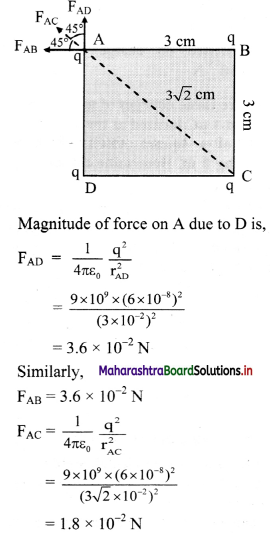∴ Resultant force on ‘A’
= FAD cos 45 + FAB cos 45 + FAC
= (3.6 × 10-2 × $$\frac {1}{√2}$$) + (3.6 × 10-2 × $$\frac {1}{√2}$$) + 1.8 × 10-2
= 6.89 × 10-2 N directed along $$\vec{F_{AC}}$$

Question 4.
The electric field in a region is given by $$\vec{E}$$ = 5.0 $$\hat{k}$$ N/C Calculate the electric flux through a square of side 10.0 cm in the following cases
i. The square is along the XY plane
ii. The square is along XZ plane
iii. The normal to the square makes an angle of 45° with the Z axis.
Given: $$\vec{E}$$ = 5.0 $$\hat{k}$$ N/C, |E| = 5 N/C
l = 10 cm = 10 × 10-2 m = 10-1 m
A = l² – 10-2
To find: Electric flux in three cases.
1) (ø2) (ø3)
Formula: (ø1) = EA cos θ
Calculation:
Case I: When square is along the XY plane,
∴ θ = 0
ø1 = 5 × 10-2 cos 0
= 5 × 10-2 V m

Case II: When square is along XZ plane,
∴ θ = 90°
ø1 = 5 × 10-2 cos 90° = 0 V m

Case III: When normal to the square makes an angle of 45° with the Z axis.
∴ 0 = 45°
∴ ø3 = 5 × 10-2 × cos 45°
= 3.5 × 10-2 V m

Question 5.
Three equal charges of 10 × 10-8 C respectively, each located at the corners of a right triangle whose sides are 15 cm, 20 cm and 25 cm respectively. Find the force exerted on the charge located at the 90° angle.
Given: qA = qB = qC = 10 × 10-8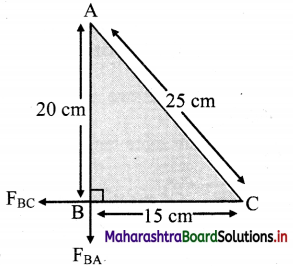Force on B due to A,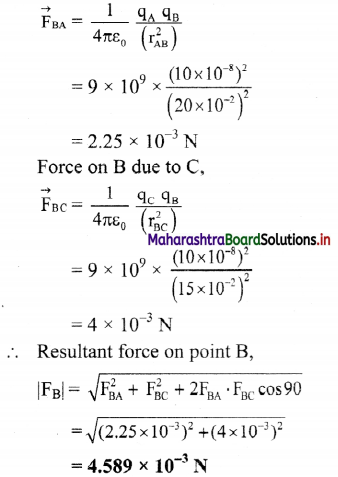Question 6.
A potential difference of 5000 volt is applied between two parallel plates 5 cm apart. A small oil drop having a charge of 9.6 x 10-19 C falls between the plates. Find (i) electric field intensity between the plates and (ii) the force on the oil drop.
Given: V = 5000 volt, d = 5 cm = 5 × 10-2 m
q = 9.6 × 10-19 C
To find:
i. Electric field intensity (E)
ii. Force (F)
Formula:
i. E = $$\frac {V}{d}$$ $$\frac {q}{r}$$
ii. E = $$\frac {F}{q}$$
Calculation: From formula (i),
E = $$\frac {F}{q}$$ = 105 N/C
From formula (ii)
F = E x q
= 105 × 9.6 × 10-19
= 9.6 × 10-14 N

Question 7.
Calculate the electric field due to a charge of -8.0 × 10-8 C at a distance of 5.0 cm from it.
Given: q = – 8 × 10-8 C, r = 5 cm = 5 × 10-2 m
To Find: Electric field (E)
Formula: E = $$\frac {1}{4πε_0}$$ $$\frac {q}{r^2}$$
Calculation: From formula,
E = 9 × 109 × $$\frac {(-8×10^{-8})}{(5×10^{-2})^2}$$
= -2.88 × 105 N/C

11th Physics Digest Chapter 10 Electrostatics Intext Questions and Answers

Can you recall? (Textbookpage no. 188)

Question 1.
Have you experienced a shock while getting up from a plastic chair and shaking hand with your friend?
Yes, sometimes a shock while getting up from a plastic chair and shaking hand with friend is experienced.

Question 2.
Ever heard a crackling sound while taking out your sweater in winter?
Yes, sometimes while removing our sweater in winter, some crackling sound is heard and the sweater appears to stick to body.

Question 3.
Have you seen the lightning striking during pre-monsoon weather?
Yes, sometimes lightning striking during pre-pre-monsoon weather seen.

Can you tell? (Textbook page no. 189)

i. When a petrol or a diesel tanker is emptied in a tank, it is grounded.
ii. A thick chain hangs from a petrol or a diesel tanker and it is in contact with ground when the tanker is moving.
i. When a petrol or a diesel tanker is emptied in a tank, it is grounded so that it has an electrically conductive connection from the petrol or diesel tank to ground (Earth) to allow leakage of static and electrical charges.

ii. Metallic bodies of cars, trucks or any other big vehicles get charged because of friction between them and the air rushing past them. Hence, a thick chain is hanged from a petrol or a diesel tanker to make a contact with ground so that charge produced can leak to the ground through chain.Can you tell? (Textbook page no. 194)

Three charges, q each, are placed at the vertices of an equilateral triangle. What will be the resultant force on charge q placed at the centroid of the triangle?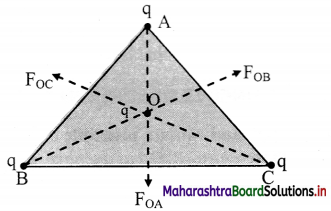Since AD. BE and CF meets at O, as centroid of an equilateral triangle.
∴ OA = OB = OC
∴ Let, r = OA = OB = OC
Force acting on point O due to charge on point A,
$$\overrightarrow{\mathrm{F}}_{\mathrm{OA}}=\frac{1}{4 \pi \varepsilon_{0}} \frac{\mathrm{q}^{2}}{\mathrm{r}_{i}^{2}} \hat{\mathrm{r}}_{\mathrm{AO}}$$
Force acting on point O due to charge on point B,
$$\overrightarrow{\mathrm{F}}_{O \mathrm{~B}}=\frac{1}{4 \pi \varepsilon_{0}} \frac{\mathrm{q}^{2}}{\mathrm{r}^{2}} \hat{\mathrm{r}}_{\mathrm{BO}}$$
Force acting on point O due to charge on point C,
$$\overrightarrow{\mathrm{F}}_{\mathrm{OC}}=\frac{1}{4 \pi \varepsilon_{0}} \frac{\mathrm{q}^{2}}{\mathrm{r}^{2}} \hat{\mathrm{r}} \mathrm{co}$$
∴ Resultant force acting on point O,
F = $$\vec{F}$$OA + $$\vec{F}$$OB + $$\vec{F}$$OC
On resolving $$\vec{F}$$OB and $$\vec{F}$$OC, we get –$$\vec{F}$$OA
i.e., $$\vec{F}$$OB + $$\vec{F}$$OC = –$$\vec{F}$$OA
∴ $$\vec{F}$$ = $$\vec{F}$$OA – $$\vec{F}$$OA = 0
Hence, the resultant force on the charge placed at the centroid of the equilateral triangle is zero.

Can you tell? (Textbook page no. 197)

Why a small voltage can produce a reasonably large electric field?

1. Electric field produced depends upon voltage as well as separation distance.
2. Electric field varies linearly with voltage and inversely with distance.
3. Hence, even if voltage is small, it can produce a reasonable large electric field when the gap between the electrode is reduced significantly.

Can you tell? (Textbook page no. 198)

Lines of force are imaginary; can they have any practical use?
Yes, electric lines of force help us to visualise the nature of electric field in a region.

Can you tell? (Textbook page no. 204)

The surface charge density of Earth is σ = -1.33 nC/m². That is about 8.3 × 109 electrons per square metre. If that is the case why don’t we feel it?